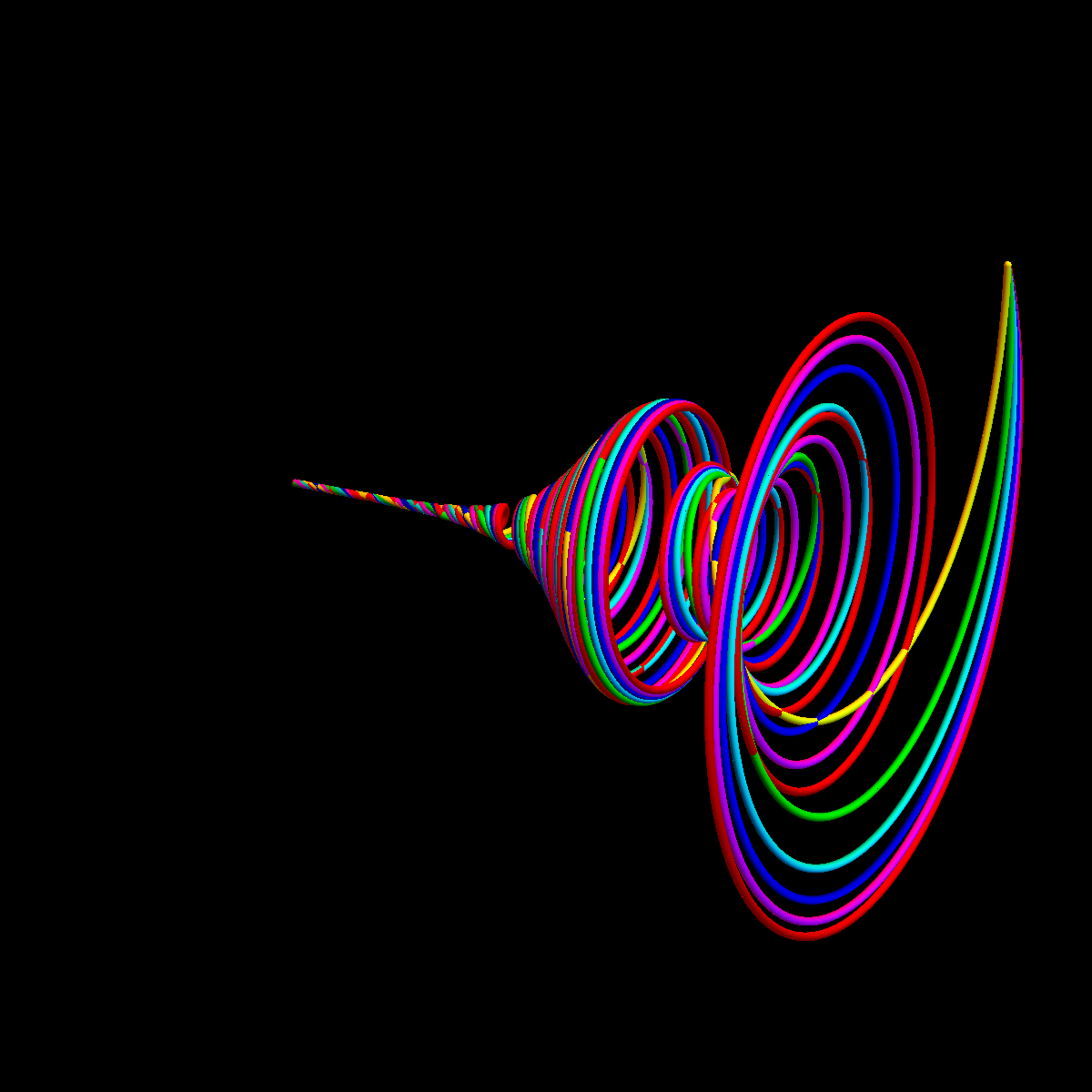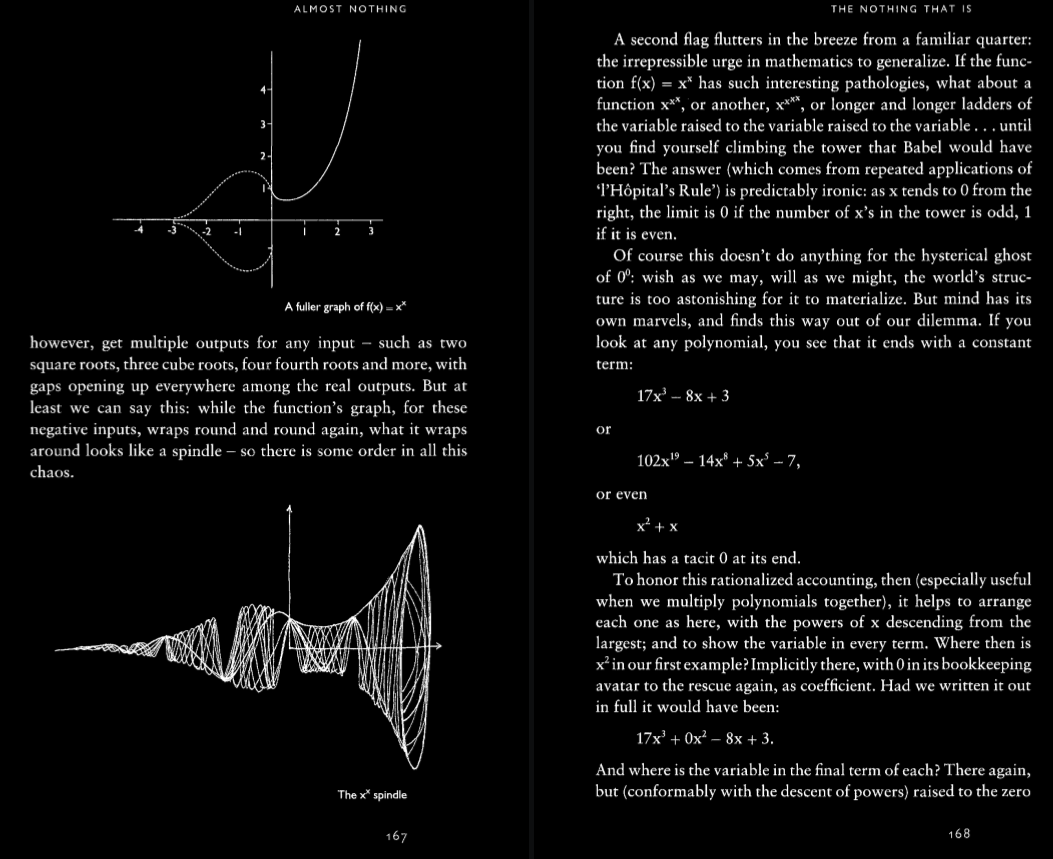The function f(x) = xx is pretty cool! One can write it as f(x) = exp(i log(x)) but since the log has complex values and is not uniquely determined, the function is actually multi valued. For any k, we get a function f(x) = exp(i (log(x) + 2 π k) which has a real and imaginary part. How can we visualize this? The best is to write it as parametrized curves r(t) = < t, Re(f(t)), Im(f(t)) >. The resulting curves define what one calls the xx spindle. Here is a picture drawn in Mathematica. We take k=1,2,3,4,5.This term can be found in the book of Robert Kaplan The nothing that is on page 167.